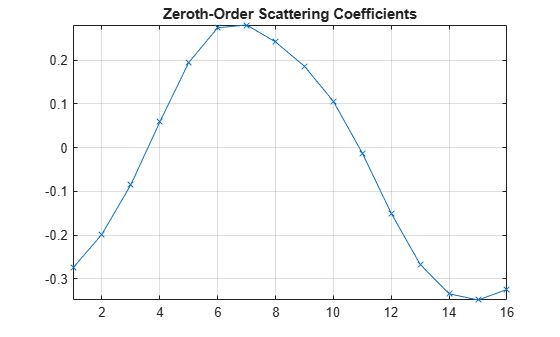# numCoefficients

Number of wavelet scattering coefficients

## Syntax

``ncf = numCoefficients(sf)``

## Description

example

````ncf = numCoefficients(sf)` returns the number of scattering coefficients for each scattering path in the wavelet time scattering network `sf`. The number of scattering coefficients depends on the values of the SignalLength, InvarianceScale, and OversamplingFactor properties of `sf`.```

## Examples

collapse all

This example shows how to oversample a 1-D wavelet scattering transform.

Load an ECG signal sampled at 180 Hz, and create a wavelet time scattering network to process the signal. To perform a critically downsampled wavelet scattering transform, do not change the value of the `OversamplingFactor` property in `sf`. Return the number of scattering coefficients for the scattering network.

```load wecg Fs = 180; sf = waveletScattering('SignalLength',numel(wecg),'SamplingFrequency',Fs); ncf = numCoefficients(sf)```
```ncf = 8 ```

Return the 1-D wavelet scattering transform of `wecg`, and plot the zeroth-order scattering coefficients. Confirm the number of zeroth-order scattering coefficients is equal to `ncf`.

```s = scatteringTransform(sf,wecg); display(['Number of zeroth-order scattering coefficients: ',... num2str(numel(s{1}.signals{1}))])```
```Number of zeroth-order scattering coefficients: 8 ```
```plot(s{1}.signals{1},'x-') grid on axis tight title('Zeroth-Order Scattering Coefficients')```To oversample the scattering coefficients by a factor of 2, set the `OversamplingFactor` property of `sf` equal to 1 (because ${\mathrm{log}}_{2}2=1$). Return the number of scattering coefficients for the edited network. Confirm the number of scattering coefficients has doubled.

```sf.OversamplingFactor = 1; ncf = numCoefficients(sf)```
```ncf = 16 ```

Return the wavelet scattering transform of `wecg` using the edited network, and plot the zeroth-order scattering coefficients. Since the number of coefficients in the critically sampled transform is equal to 8, confirm that the number of zeroth-order coefficients in the oversampled transform is equal to 16.

```s = scatteringTransform(sf,wecg); figure plot(s{1}.signals{1},'x-') grid on axis tight title('Zeroth-Order Scattering Coefficients')```## Input Arguments

collapse all

Wavelet time scattering network, specified as a `waveletScattering` object.

## Extended Capabilities

### C/C++ Code GenerationGenerate C and C++ code using MATLAB® Coder™.